# Engineering Mechanics - KOP: Impulse and Momentum

## Why Engineering Mechanics KOP: Impulse and Momentum?

In this section you can learn and practice Engineering Mechanics Questions based on "KOP: Impulse and Momentum" and improve your skills in order to face the interview, competitive examination and various entrance test (CAT, GATE, GRE, MAT, Bank Exam, Railway Exam etc.) with full confidence.

## Where can I get Engineering Mechanics KOP: Impulse and Momentum questions and answers with explanation?

IndiaBIX provides you lots of fully solved Engineering Mechanics (KOP: Impulse and Momentum) questions and answers with Explanation. Solved examples with detailed answer description, explanation are given and it would be easy to understand. All students, freshers can download Engineering Mechanics KOP: Impulse and Momentum quiz questions with answers as PDF files and eBooks.

## Where can I get Engineering Mechanics KOP: Impulse and Momentum Interview Questions and Answers (objective type, multiple choice)?

Here you can find objective type Engineering Mechanics KOP: Impulse and Momentum questions and answers for interview and entrance examination. Multiple choice and true or false type questions are also provided.

## How to solve Engineering Mechanics KOP: Impulse and Momentum problems?

You can easily solve all kind of Engineering Mechanics questions based on KOP: Impulse and Momentum by practicing the objective type exercises given below, also get shortcut methods to solve Engineering Mechanics KOP: Impulse and Momentum problems.

### Exercise :: KOP: Impulse and Momentum - General Questions

• KOP: Impulse and Momentum - General Questions
1.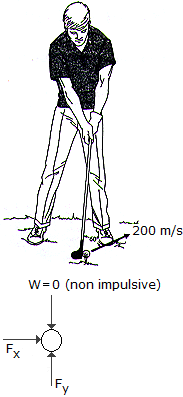A golf ball having a mass of 40 g is struck such that it has an initial velocity of 200 m/s as shown. Determine the horizontal and vertical components of the impulse given to the ball.

 A. Impx = 4.00 N-s, Impy = 6.93 N-s B. Impx = 6.93 N-s, Impy = 4.00 N-s C. Impx = 6.93 N-s, Impy = 4.39 N-s D. Impx = 4.00 N-s, Impy = 7.32 N-s

Explanation:

No answer description available for this question. Let us discuss.

2.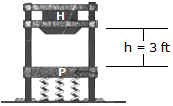The drop hammer H has a weight of 900 lb and falls from rest. H has a weight of 900 lb and falls from rest h = 3 ft onto a forged anvil plate P that has a weight of 500 lb. The plate is mounted on a set of springs which have a combined stiffness of kT = 500 lb/ft. Determine (a) the velocity of P and H just after collision and (b) the maximum compression in the springs caused by the impact. The coefficient of restitution between the hammer and the plate is e = 0.6. Neglect friction along the vertical guide posts A and B.

 A. vH2 = 3.97 ft/s, vP2 = 17.87 ft/s, x = 4.15 ft B. vH2 = 8.94 ft/s, vP2 = 8.94 ft/s, x = 2.57 ft C. vH2 = 5.96 ft/s, vP2 = 14.30 ft/s, x = 3.52 ft D. vH2 = 20.8 ft/s, vP2 = 12.51 ft/s, x = 3.20 ft

Explanation:

No answer description available for this question. Let us discuss.

3.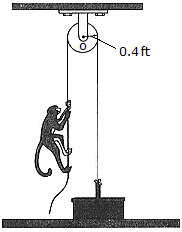A basket and its contents weigh 10 lb. A 20-lb monkey grabs the other end of the rope and very quickly (almost instantaneously) accelerates by pulling hard on the rope until he is moving with a constant speed of vm/r = 2 ft/s measured relative to the rope. The monkey then continues climbing at this constant rate relative to the rope for 3 seconds. How fast is the basket rising at the end of the 3 seconds? Neglect the mass of the pulley and the rope.

Explanation:

No answer description available for this question. Let us discuss.

4.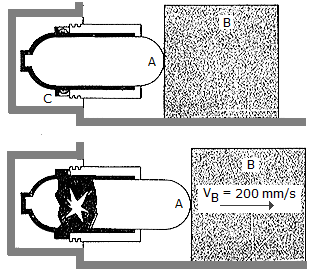In cases of emergency, the gas actuator can be used to move a 75-kg block B by exploding a charge C near a pressurized cylinder of negligible mass. As a result of the explosion, the cylinder fractures and the released gas forces the front part ofthe cylinder, A, to move B and the floor is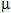= 0.5, determine the impulse that the actuator must impart to B.

 A. Imp = 147.2 N-s B. Imp = 132.2 N-s C. Imp = 15.00 N-s D. Imp = 162.2 N-s

Explanation:

No answer description available for this question. Let us discuss.

5.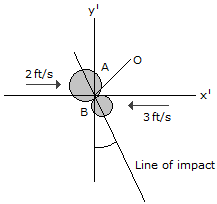Two coins A and B have the initial velocities shown just before they collide at point O. If they have weights of WA = 13.2(10-3) lb and WB = 6.6(10-3) lb and the surface upon which they slide is smooth, determine their speed just after impact. The coefficient of restitution is e = 0.65.

 A. vA2 = 1.856 ft/s, vB2 = 3.18 ft/s B. vA2 = 1.871 ft/s, vB2 = 2.76 ft/s C. vA2 = 1.772 ft/s, vB2 = 2.88 ft/s D. vA2 = 1.755 ft/s, vB2 = 2.92 ft/s